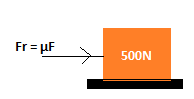mycollegehive
A 500N box rests on a horizontal floor. A constant horizonta...
physics waec-physics-2020 ssce-exam-2020 waec-20200A 500N box rests on a horizontal floor. A constant horizontal force is exerted on the box so that it moves through 8m. If the coefficient of kinetic friction between the floor and the box is 0.22, calculate the work done on the box.

A. 880J

B. 440J

C. 400J

D. 110J

397 viewsShareFollowUniversity of Lagos Nigeria
29 August 2020School not set Nigeria
23 October 20200Fr is the force applied on the box which causes it to move.

F is the force exerted by th box

μ is the coefficient of friction.

Fr = 0.22 x 500

= 110N

Therefore, the work done, W = Fr x d

where,

Fr is the force applied on the box which causes it to move and d is the distance moved

W = 110 x 8

= 880JShare

### Groups

How to insert math formulas/equations### Related Tags

physics

6 followers

121 questionswaec-physics-2020

7 followers

48 questionsssce-exam-2020

10 followers

273 questionswaec-2020

12 followers

273 questions